Part 1 summarised how I started in FP in C# by learning LINQ and trying Project Euler puzzles.
Part 2 is this article taking a detailed look at the first Euler puzzle with source for the first puzzle and source code for the next 17 puzzles using Imperative and LINQ
Part 3 is making C# code more functional by using abstractions from the excellent functional c# library
Notes Are my developer notes whilst working through the bookWhat is projecteuler.net?

From Wikipedia:
Named after the 18th Century Swiss mathematician, widely considered the most prolific mathematician of all time.

The website is a series of problems intended to be solved with computer programs

Since its creation in 17 years ago, a new problem has been added every 2 weeks giving a total of 600 problems.

There are more than 800,000 users from all over the world who have solved 1 problem.

I’ve been using this site over the last 7 years to learn new languages, and to improve my skills in existing languages

The first question is:

If we list all the natural numbers below 10 that are multiples of 3 or 5, we get 3, 5, 6 and 9. The sum of these multiples is 23.
Find the sum of all the multiples of 3 or 5 below 1000.

Visually this looks like: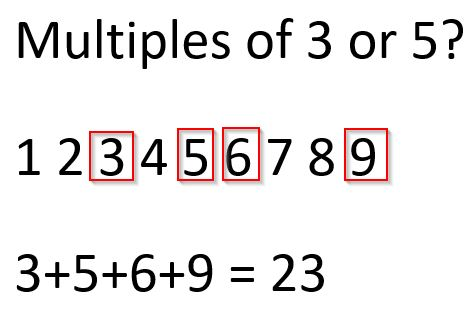My strategy is always to

• Get something working
• Check the solution is correct
• Improve the solution

## 1. First try - Imperative approach

Lets try this using the latest version (10th Oct 2018) of the .NET Core runtime which is 2.1, which includes the C# language which is version 7.3. Download for Win / Mac.

Source code for this Euler1 problem is on Github.

``````private static void Main() => Console.WriteLine(\$"Answer is: {Run(10)}");

// 1. Imperative approach. Take every number from 1..number and see if it is divisible by 3 or 5
private static int Run(int number)
{
int total = 0;
for (var i = 1; i < number; i++)
if (i % 3 == 0 || i % 5 == 0)
total += i;
}
``````

Once you have a program that works, lets see if the answer is correct! Firstly you’ll need to sign up then: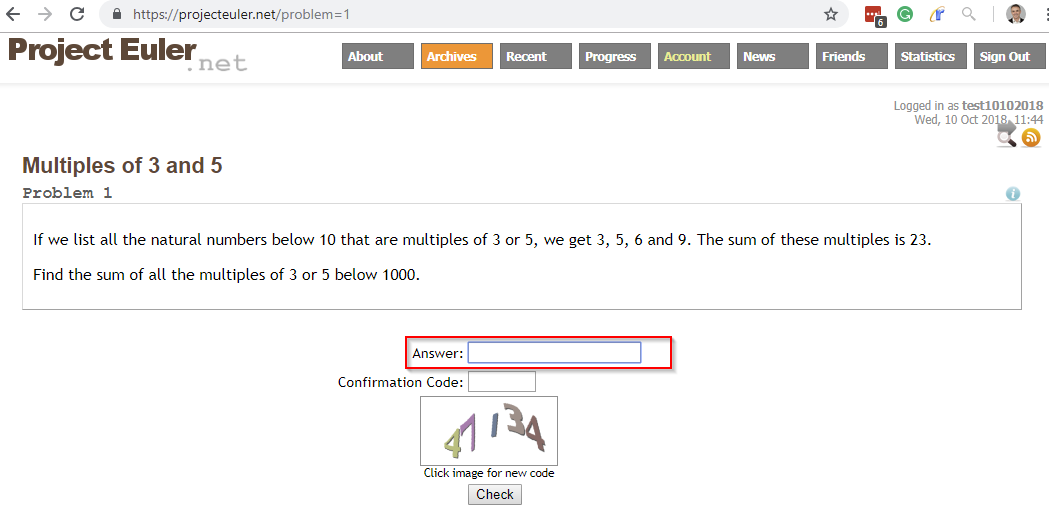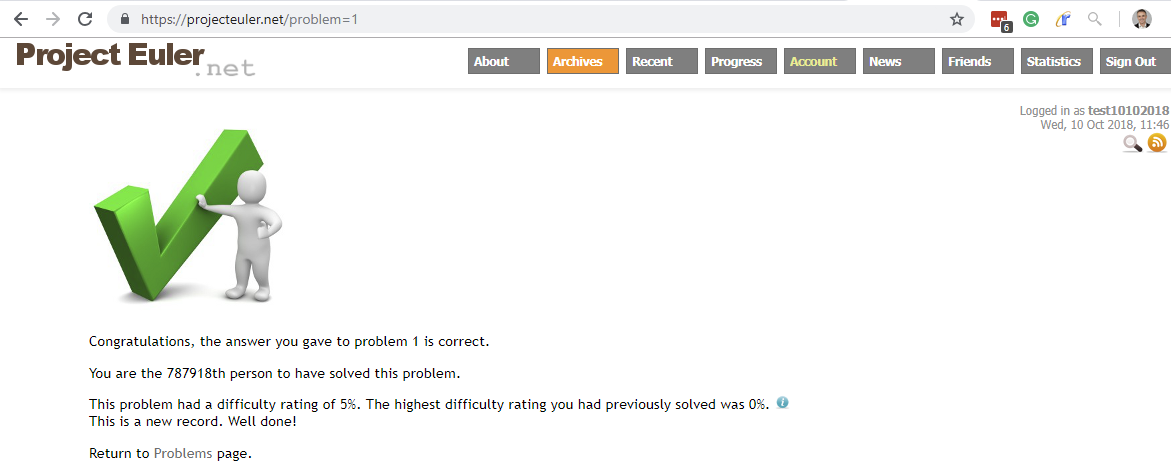Once you do a few problems, this is a gratifying sight. It is worth mentioning the Project Euler do not like example code posted on the web (and public repositories) as this deprives others of the chance to the solve the problems by themselves.

Interesting points to consider here are:

• Expression Body Member usage in Main method
• String interpolation in Main
• Not using brackets in Run method

## 2. Second Try - Improvement! - Functional approach

The point of this post is how to improve! - so now I have working code lets try a different approach I am interested in Functional Programming, and on this, my fifth time going through some Euler puzzles I’m pushing in that direction. LINQ Language Integrated Query introduced in .NET3.5 in 2007 is a very functional set of concepts.

``````private static int RunLinq(int n) => Range(1, n - 1).Where(x => x % 3 == 0 || x % 5 == 0).Sum();
``````
• Create a Range
• Pass a Lambda expression which is a Predicate (a function which takes parameter(s) and returns a bool)
• Finally using the Sum extension method

I love this syntax (now I’ve been using it for a while) as it expresses concisely what the code is doing.

### TDD with xUnit

I find Euler problems lead themselves to easy unit testing and TDD. This is especially useful when trying new ways solving a problem.

I use xUnit with Visual Studio. Firstly install xUnit and then the test runner.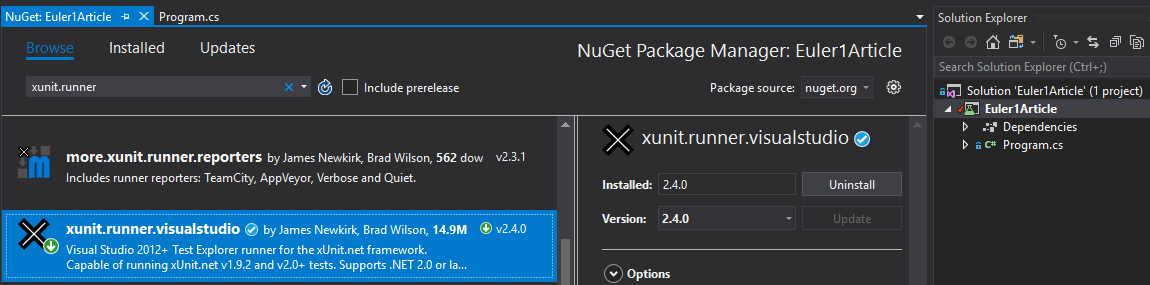then solve the Error:

“Program has more than one entry point defined”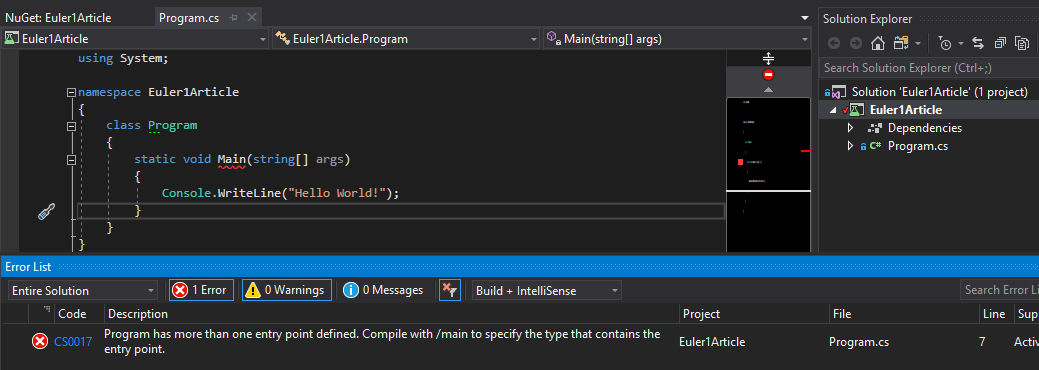Detail but essentially:

• Edit the csproj file (right click on the project in visual studio)
• Add 1 line.. ‘GenerateProgramFile false GenerateProgramFile’ so the csproj file should look like:
``````<Project Sdk="Microsoft.NET.Sdk">
<PropertyGroup>
<OutputType>Exe</OutputType>
<TargetFramework>netcoreapp2.1</TargetFramework>
<GenerateProgramFile>false</GenerateProgramFile>
</PropertyGroup>

<ItemGroup>
<PackageReference Include="xunit" Version="2.4.0" />
<PackageReference Include="xunit.runner.visualstudio" Version="2.4.0">
<PrivateAssets>all</PrivateAssets>
<IncludeAssets>runtime; build; native; contentfiles; analyzers</IncludeAssets>
</PackageReference>
</ItemGroup>
</Project>
``````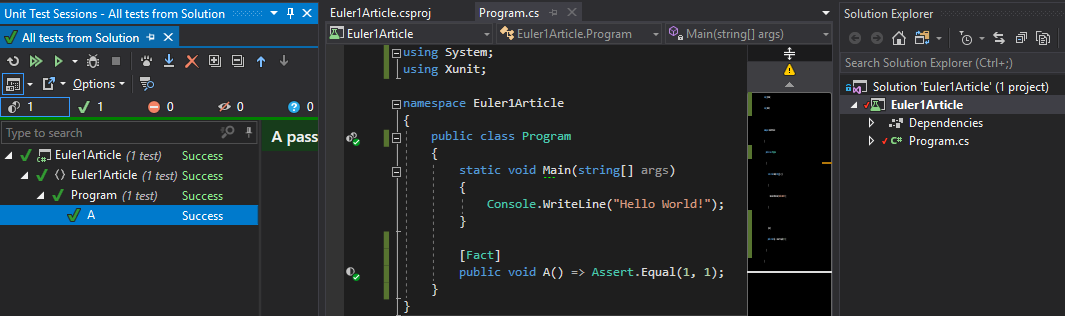Successfully test your test runner (I use the ReSharper test runner)

### TDD Euler1

Euler problems commonly have a test case example which can be a good unit test ie:

If we list all the natural numbers below 10 that are multiples of 3 or 5, we get 3, 5, 6 and 9. The sum of these multiples is 23.
Find the sum of all the multiples of 3 or 5 below 1000.

So a good unit test will be:

``````[Fact]
public void RunTest() => Assert.Equal(23, Run(10));
``````

then I can write an implementation:

``````private static int Run(int number)
{
int total = 0;
for (var i = 1; i < number; i++)
if (i % 3 == 0 || i % 5 == 0)
total += i;
}
``````

## Refactoring tools

I use Visual Studio and ReSharper to explore different ways to express code. In this example R# helped me discover:

• Expression body member
• Brackets
• Import static member for Enumerable

## Summary

Project Euler is a great way to improve your programming skills by solving, refactoring and exploring different approaches. I particularly enjoyed in this problem TDD and LINQ.

Why not have some fun go and do a problem now :-)